# Excel DAX - Calculated Fields / Measures

A calculated field in a table in a Data Model is the field obtained by a DAX formula. In earlier versions of Power Pivot, the calculated field was termed as a measure. In Excel 2013, it was renamed as a calculated field. However, it is renamed back to measure in Excel 2016. If you refer to any documentation, you can observe a mix up of these two terms. Note that the terms calculated field and measure are synonymous. In this tutorial, we use the term calculated field.

## Understanding Calculated Fields

A calculated field is a formula that is created specifically for use in a PivotTable (or PivotChart).

You can create a calculated field based on standard aggregation functions, such as COUNT or SUM, or by defining your own DAX formula.

Following is the difference between the calculated field and the calculated column −

• A calculated field can be used only in the VALUES area of a PivotTable.

• A calculated column with the calculated results can be used in ROWS, COLUMNS and FILTERS areas also.

### Saving Calculated Field

The calculated field will be saved with its source table in the Data Model. It appears in the Power PivotTable or Power PivotChart Fields list as a field in the table.

### Using Calculated Field

To use a calculated field, you have to select it from the Power PivotTable Fields list. The calculated field will get added to the VALUES area and the formula used for the calculated field will be evaluated. A result is created for each combination of row and column fields.

## Calculated Field – An Example

Consider the following Data Model for Olympics data −As seen in the above screenshot, the Results table has a field Medal that contains the values – Gold, Silver, or Bronze for each of the rows containing Sport – Event - Country – Date combination. Suppose you want medal count for each country, then you can create a calculated field Medal Count with the following DAX formula −

```Medal Count := COUNTA([Medal])
```

## Creating a Calculated Field in a Table

To create the calculated field Medal Count in the Results table, do the following −

• Click the cell in the calculation area below the Medal column in the Results table. The cell will be highlighted.

• Type Medal Count:=COUNTA([Medal]) in the formula bar.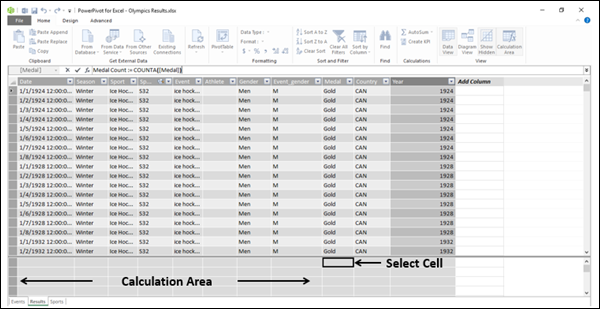Press Enter.As seen in the above screenshot, the calculated field appears in the selected cell, showing the value as 34,094. This number is the total number of rows in the Results table. Hence, it does not make much sense at the first look. As discussed earlier, the real use of a calculated field can be seen only by adding it to a Power PivotTable or a Power PivotChart.

## Using the Calculated Field in a Power PivotTable

To use the calculated field to count the number of medals for each country, do the following −

• Click the PivotTable on the Ribbon in the Power Pivot window.
• Click the PivotTable in the dropdown list.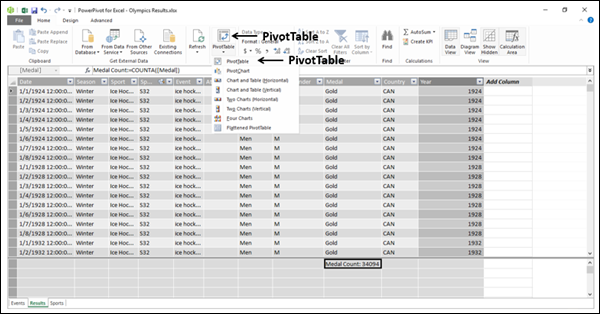Create PivotTable dialog box appears.

• Click the Existing Worksheet.
• Select where you want to place the PivotTable.

An empty PivotTable will get created.

• Click the Results table in the PivotTable Fields list.
• Click the fields – Country and Medal count.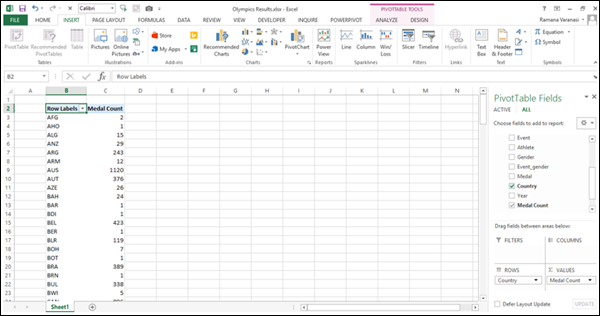As you can observe, Medal Count is added to VALUES area and Country is added to ROWS area. The PivotTable is created with the field Country values appearing in the rows. And for each row, the Medal Count value is calculated and displayed. That is the way, the calculated field evaluates the DAX formula used and displays the values.

• Add the field Sport from the Results table to ROWS area.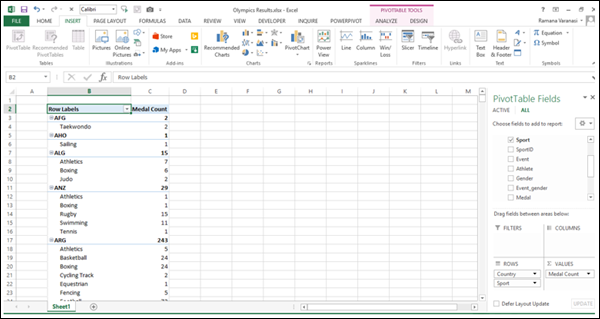As you can see in the above screenshot, Medal Count is calculated for each Country - Sport-wise and a Subtotal for the Country itself.

This is how DAX supplements the Power features.

## Types of Calculated Fields

There are two types of Calculated Fields – Implicit and Explicit.

• An implicit calculated field is created in the Power PivotTable Fields list pane.

• An explicit calculated field is created either in the table in the Power Pivot window, or from the PowerPivot Ribbon in the Excel window.

## Creating an Implicit Calculated Field

An implicit calculated field can be created in two ways, both in the Power PivotTable Fields pane.

### Creating an Implicit Calculated Field in the PivotTable Fields List

You can create the Count of Medal Field from the Medal field in the PivotTable Fields list as follows −

• Deselect the field Medal Count.
• Right-click on the field Medal.
• Click Add to Values in the dropdown list.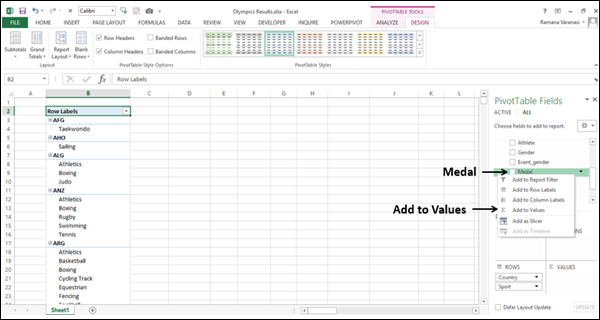Count of Medal appears in the Values area. Count of Medal column will be added to the PivotTable.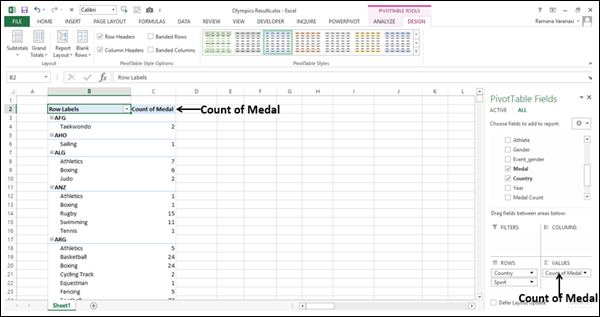### Creating an Implicit Calculated Field in the VALUES Area

You can create an implicit calculated field - % of Parent Row in the Values area to express the Medal count of each sport that a country has won as a percentage of the total number of Medals won by that Country.

• Click the down arrow in the Count of Medal box in VALUES area.
• Click the Value Field Settings in the dropdown list.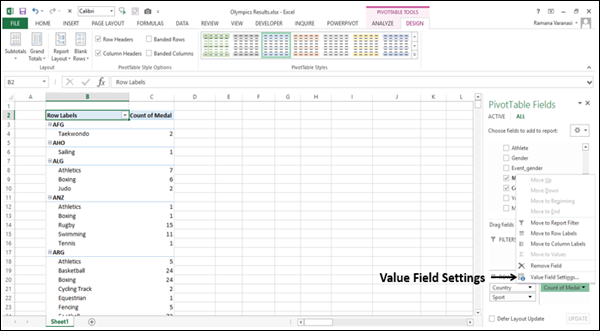Value Field Settings dialog box appears.

• Type % Medals in the Custom Name box.
• Click the Show Values As tab.
• Click the box under Show values as.
• Click the % of Parent Row Total.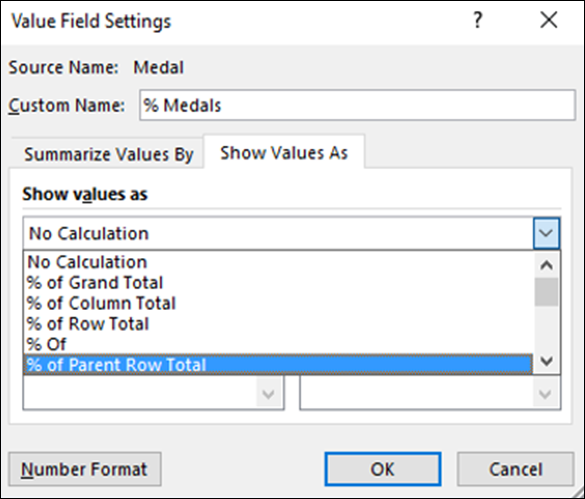• Click the Number Format button.

Format Cells dialog box appears.

• Click Percentage.
• Type 0 in decimal places.
• Click OK.
• Click OK in the Value Field Settings dialog box.
• Select Do Not Show Subtotals.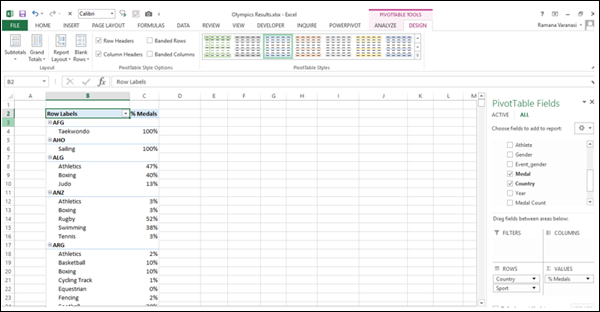You created another implicit calculated field % Medals and as you can observe, for each Country, the percentage of Medals Sport-wise are displayed.

## Drawbacks of an Implicit Calculated Field

Implicit calculated fields are easy to create. In fact, you have been creating them even in Excel PivotTables and Pivot Charts. But, they have the following drawbacks −

• They are volatile. That means, if you deselect the field you used for calculated field, it will be removed. If you want to display it again, you have to once again create it.

• Their scope is limited to the PivotTable or PivotChart in which they are created. If you create another PivotTable in another worksheet, you have to create the calculated field again.

On the other hand, explicit calculated fields will get saved with the table and will be available whenever you select that table.

## Creating an Explicit Calculated Field

You can create an explicit calculated field in two ways −

• In the calculation area in a Table in the Data Model. You have already learnt this in the section – Creating Calculated Field in a Table.

• From PowerPivot Ribbon in the Excel table. You will learn this way of creating an explicit calculated field in the next section.

### Creating an Explicit Calculated Field from PowerPivot Ribbon

To create an explicit calculated field from PowerPivot Ribbon, do the following −

• Click the POWERPIVOT tab on the Ribbon in your workbook.
• Click the Calculated Fields in the Calculations area.
• Click the New Calculated Field in the dropdown list.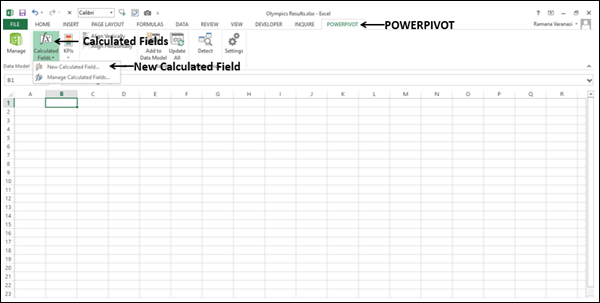Calculated Field dialog box appears.

• Fill in the required information as shown in the following screenshot.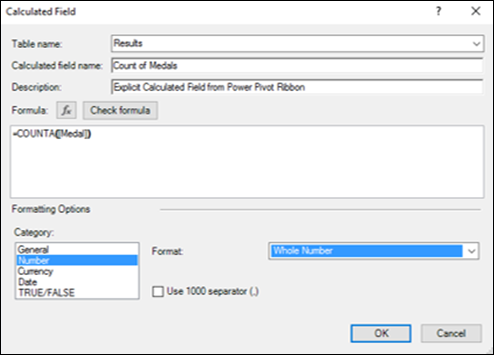• Click the Check formula button.
• Click OK only if there are no errors in the formula.

As you can observe, you can define the category and format of the calculated field in this dialog box. Further, you can use the IntelliSense feature to understand the usage of the functions and to use the AutoComplete feature to easily complete the names of the functions, tables, and columns. For details on IntelliSense feature, refer to the chapter – DAX Formulas.

This is a recommended way to create explicit calculated fields.